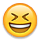# Effect of 2 panels in series

I'm still trying to understand the TLIs and FLIs (Two/Three and Four/Five Letter Initialisms) associated with solar equipment.

I have a panel with the following specs:
Voc = 45 V
Isc = 5.5 A
Pmax = 190 W
Vmp = 36 V
Imp = 5.0 A

If I connect two of them in series, assuming I'm operating at the standard conditions and dealing theoretically rather than real world, in theory should the two panels just follow Ohm's law?

i.e. For 2 in series the array would have these specs?
Voc = 90 V
Isc = 5.5 A
Pmax = 380 W
Vmp = 72 V
Imp = 5.0 A

i.e. the voltages and pmax double but the currents remain the same?

• Solar Expert Posts: 3,009 ✭✭✭✭
Re: Effect of 2 panels in series
aparker wrote: »
I'm still trying to understand the TLIs and FLIs (Two/Three and Four/Five Letter Initialisms) associated with solar equipment.

I have a panel with the following specs:
Voc = 45 V
Isc = 5.5 A
Pmax = 190 W
Vmp = 36 V
Imp = 5.0 A

If I connect two of them in series, assuming I'm operating at the standard conditions and dealing theoretically rather than real world, in theory should the two panels just follow Ohm's law?

i.e. For 2 in series the array would have these specs?
Voc = 90 V
Isc = 5.5 A
Pmax = 380 W
Vmp = 72 V
Imp = 5.0 A

i.e. the voltages and pmax double but the currents remain the same?

Welcome to the forum! And to answer your question - - YES! You have it right!
• Re: Effect of 2 panels in series

Many thanks for confirming that and for your kind welcome• Banned Posts: 17,615 ✭✭
Re: Effect of 2 panels in series

That's why we have the glossary of terms: http://forum.solar-electric.com/showthread.php?6136-Glossary• Solar Expert Posts: 1,973 ✭✭✭
Re: Effect of 2 panels in series
aparker wrote: »
I'm still trying to understand the TLIs and FLIs (Two/Three and Four/Five Letter Initialisms) associated with solar equipment.

I have a panel with the following specs:
Voc = 45 V
Isc = 5.5 A
Pmax = 190 W
Vmp = 36 V
Imp = 5.0 A

If I connect two of them in series, assuming I'm operating at the standard conditions and dealing theoretically rather than real world, in theory should the two panels just follow Ohm's law?

i.e. For 2 in series the array would have these specs?
Voc = 90 V
Isc = 5.5 A
Pmax = 380 W
Vmp = 72 V
Imp = 5.0 A

i.e. the voltages and pmax double but the currents remain the same?
Correct. Question #2:What happens when you put the same two modules (panels) in parallel?
• Banned Posts: 17,615 ✭✭
Re: Effect of 2 panels in series